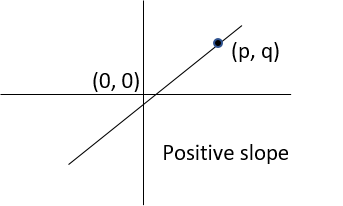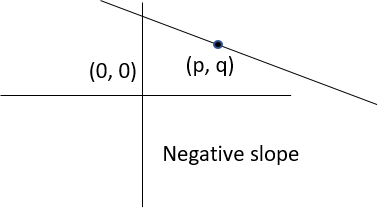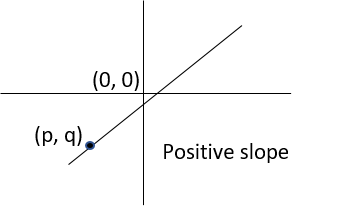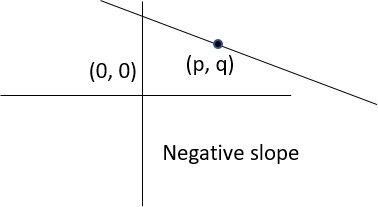# GMAT Questions | Coordinate Geometry Q5

#### Lines with positive slope, intercepts, quadrants | Data Sufficiency

This GMAT data sufficiency (DS) question is from the topic Coordinate Geometry. A medium level difficulty question testing concepts about positive sloping lines, intercepts of such lines and the quadrants through which the line passes. An interesting GMAT 650 to 700 level Co geo data sufficiency question.

This data sufficiency problem consists of a question and two statements, labeled (1) and (2), in which certain data are given. You have to decide whether the data given in the statements are sufficient for answering the question. Using the data given in the statements, plus your knowledge of mathematics and everyday facts (such as the number of days in a leap year or the meaning of the word counterclockwise), you must indicate whether -

1. Statement (1) ALONE is sufficient, but statement (2) alone is not sufficient to answer the question asked.
2. Statement (2) ALONE is sufficient, but statement (1) alone is not sufficient to answer the question asked.
3. BOTH statements (1) and (2) TOGETHER are sufficient to answer the question asked, but NEITHER statement ALONE is sufficient to answer the question asked.
5. Statements (1) and (2) TOGETHER are NOT sufficient to answer the question asked, and additional data specific to the problem are needed.

#### Numbers

All numbers used are real numbers.

#### Figures

A figure accompanying a data sufficiency question will conform to the information given in the question but will not necessarily conform to the additional information given in statements (1) and (2)

Lines shown as straight can be assumed to be straight and lines that appear jagged can also be assumed to be straight

You may assume that the positions of points, angles, regions, etc. exist in the order shown and that angle measures are greater than zero.

All figures lie in a plane unless otherwise indicated.

#### Note

In data sufficiency problems that ask for the value of a quantity, the data given in the statement are sufficient only when it is possible to determine exactly one numerical value for the quantity.

Question 5: Is the slope of the line that passes through the point (p, q) positive?

Statement 1: p, q > 0
Statement 2: The x-intercept of the line is k, such that k > p.

## GMAT Live Online Classes

### Explanatory Answer | GMAT Coordinate Geometry DS

#### Step 1: Decode the Question Stem

Q1. What kind of an answer will the question fetch?
The question is an "Is" question. Answer to an "is" questions is either YES or NO.

Q2. When is the data sufficient?
If we get a conclusive yes, the data is sufficient. The data is sufficient even if we get a conclusive no. The data is not sufficient only when we do not get a conclusive answer.

Q3. When is the answer YES and when is it NO?
If we can determine that the slope of the line is positive, the answer is yes.
Conversely, if we can determine that the slope is either negative or is 0, the answer is no.

#### Step 2: Evaluate Statement 1 ALONE

Statement 1: p, q > 0

From this information, we know that the point (p, q) is a point in the first quadrant. Both positive and negative sloping lines pass through the first quadrant as shown below.We are NOT able to find a DEFINITE answer to the question using Statement 1.
Hence, statement 1 alone is NOT sufficient.
Eliminate answer options A and D.

#### Step 3: Evaluate Statement 2 ALONE

Statement 2: The x-intercept of the line is k, such that k > p.

We do not know anything about p. So, the line could either be positive sloping or negative sloping depending on what value p takes.

Approach: Look for a counter example
Example: The point (p, q) is a third quadrant point. The x intercept of the line is greater than p. The line has a positive slope.Counter Example: The point (p, q) is a first quadrant point. The x intercept of the line is greater than p. The line has a negative slope.We are unable to answer the question with a DEFNITE YES/NO using Statement 2.
Hence, statement 2 alone is NOT sufficient.

#### Step 4: Evaluate Statements TOGETHER

Statement 1: p, q > 0
Statement 2: The x-intercept of the line is k, such that k > p.

A line with a positive slope will have both its x and y coordinates increasing simultaneously.

The y coordinate of the x-intercept of the line is 0. The y coordinate of any point (p, q) in the first quadrant will be positive. So, q > 0.

For positive sloping lines, because x and y coordinates must increase in tandem, if point (p, q) is in the first quadrant its x coordinate will be greater than the x – intercept. So, p > k.
From statement 2, we know that p < k. So, the line CANNOT be positive sloping.

We are able to find a conclusive answer to the question.
Hence, statements TOGETHER are SUFFICIENT.

#### GMAT Online CourseTry it free!

Register in 2 easy steps and
Start learning in 5 minutes!

#### GMAT Live Online Classes

Next Batch June 18, 2023

##### Where is Wizako located?

Wizako - GMAT, GRE, SAT Prep
An Ascent Education Initiative
14B/1 Dr Thirumurthy Nagar 1st Street
Nungambakkam
Chennai 600 034. India

Work @ Wizako

##### How to reach Wizako?

Mobile: (91) 95000 48484
WhatsApp: WhatsApp Now
Email: learn@wizako.com